Courses

# Past Year Questions: Shear Force and Bending Moment Notes | EduRev

## Civil Engineering (CE): Past Year Questions: Shear Force and Bending Moment Notes | EduRev

The document Past Year Questions: Shear Force and Bending Moment Notes | EduRev is a part of the Civil Engineering (CE) Course Topic wise GATE Past Year Papers for Civil Engineering.
All you need of Civil Engineering (CE) at this link: Civil Engineering (CE)

Q.1 A simply supported beam is subjected to a uniformly distributed load. Which one of the following statements is true?    [2017 : 1 Mark, Set-I]
(a) Maximum or minimum shear force occurs where the curvature is zero.
(b) Maximum or minimum bending moment occurs where the shear force is zero.
(c) Maximum or minimum bending moment occurs where the curvature is zero.
(d) Maximum bending moment and maximum shear force occur at the same section.
Ans.
(B)

Q.2 Consider the three prismatic beams with the clamped supports P, Q and R as shown in the figures.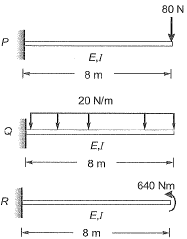Given that the modulus of elasticity, E is 2.5 x 104 MPa; and the moment of inertia, I is 8 x 108 mm4, the correct comparison of the magnitudes of the shear force Sand the bending moment M developed at the supports is    [2017 : 2 Marks, Set-II]
(a) SP < SQ < SR: MP = MQ - MR
(b) SP = SQ < SR: MP = MQ > MR
(c) SP < SQ > SR: MP = MQ = MR
(d)  SP < SQ < SR: MP < MQ < MR
Ans.
(C)
Solution: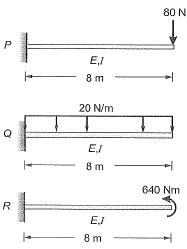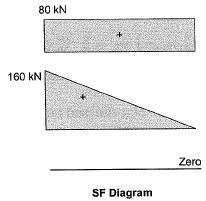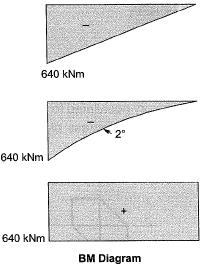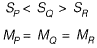Hence correct option is (c).

Q.3 A rigid member ACB is shown in the figure. The member is supported at A and B by pinned and guided roller supports, respectively. A force Pacts at Cas shown. Let RAh and RBh be the horizontal reactions at supports A and B, respectively, and RAv be the vertical reaction at support A. Selfweight of the member may be ignored.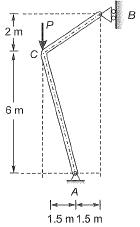Which one of the following sets gives the correct magnitudes of RAv, RBh and RAv?    [2016 : 2 Marks, Set-I]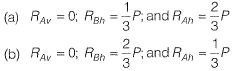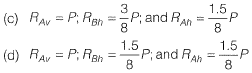Ans.
(D)
Solution: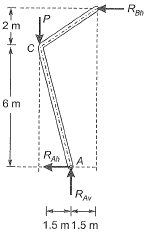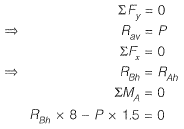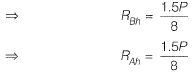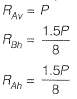Q.4 The following statement are related to bending of beams
I. The slope of the bending moment diagram is equal to the shear force.
II. The slope of the shear force diagram is equal to the load intensity.
III. The slope of the curvature is equal to the fiexural rotation.
IV. The second derivative of the deflection is equal to the curvature.
The only FALSE statement is    [2012 : 1 Mark]
(a) I
(b) II
(c) III
(d) IV
Ans.
(C)
Solution:
We know that,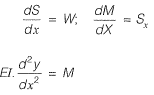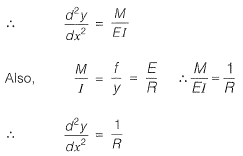Q.5 For the cantilever bracket, PQRS, loaded as shown in the a djoining figure ( PQ = RS = L, and QR = 2L), which of the following statements is FALSE?    [2011 : 2 Marks]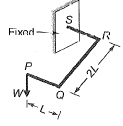(a) The portion RS has a constant twisting moment with a value of 2WL.
(b) The portion QR has a varying twisting moment with a maximum value of WL.
(c) The portion PQ has a varying bending moment with a maximum value of WL.
(d) The portion PQ has no twisting moment,
Ans.
(B)
Solution: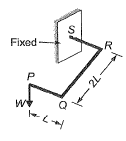Bending moment at a distance x from P, M= Wx Member PQ has varying BM with a maximum value of WL at Q and it has no twisting moment.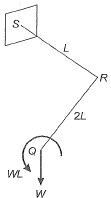Now moment WL will act as a twisting moment for the member QR. Portion QPhas varying BM with a maximum value of 2WL at R.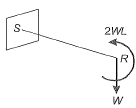Now moment 2 Wl will act as a twisting moment for portion RS which is constant in nature.

Q.6 For the simply supported beam of length L, subjected to a uniformly distributed moment M kN-m per unit length as shown in the figure, the bending moment (in kN-m) at the mid-span of the beam is    [2010 : 2 Marks]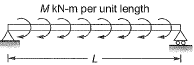(a) Zero
(b) M
(c) ML
(d) M/L
Ans.
(A)
Solution:
Let the reaction at the right hand support be VR upwards. Taking moments about left hand support, we get,
VR x L - ML = 0 ⇒ VR = M
Thus, the reaction at the left hand support VL will be M downwards.
∴ Moment at the mid-span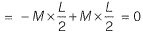Infact the bending moment through out the beam is zero.

Q.7 Two people weighing Weach are sitting on a plank of length L floating on water at L/4 from either end. Neglecting the weight of the plank, the bending moment at the centre of the plank is    [2010 : 1 Mark]
(a) WL/8
(b) WL/16
(c) WL/32
(d) zero
Ans.
(D)
Solution:
The plank will be balanced by the buoyant force acting under its bottom. Let the intensity of buoyant force be w.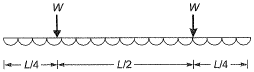For equilibrium, w x L = W + W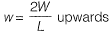Thus, the bending moment at the centre of the plank will be,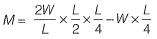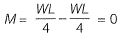The document Past Year Questions: Shear Force and Bending Moment Notes | EduRev is a part of the Civil Engineering (CE) Course Topic wise GATE Past Year Papers for Civil Engineering.
All you need of Civil Engineering (CE) at this link: Civil Engineering (CE)Use Code STAYHOME200 and get INR 200 additional OFF Use Coupon Code
All Tests, Videos & Notes of Civil Engineering (CE): Civil Engineering (CE)

### Top Courses for Civil Engineering (CE)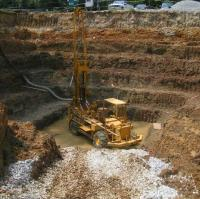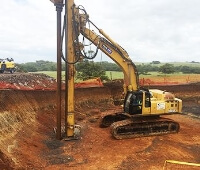## Topic wise GATE Past Year Papers for Civil Engineering

67 docs

### Top Courses for Civil Engineering (CE)Track your progress, build streaks, highlight & save important lessons and more!

,

,

,

,

,

,

,

,

,

,

,

,

,

,

,

,

,

,

,

,

,

;